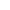###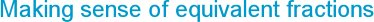Monday, 1 October 2018
Being able to recognise and manipulate equivalent fractions is a key concept when working with fractions and an essential step towards ordering and calculating with fractions successfully. I find that children use models and images well to represent equivalent fractions, particularly with fraction bars. However, from observing lessons, the biggest issue seems to be a weak basic understanding of the numerator and the denominator and a lack of fluency when it comes to equivalence and proportionality.

The language you use is important when showing the parts of a fraction.
In my Collins Maths Dictionary I explain it as follows:

The denominator shows you the number of equal parts that a whole shape or number is divided into. The numerator tells you how many of these equal parts you are using.

This can then be modelled using cubes or rods and shown as a diagram while using the language, then repeated for different fractions of a whole shape. It is important to keep the whole shape the same so that the equal parts of each can be compared.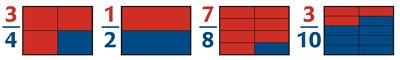Once children have a good feel for the meaning and purpose of each part of a fraction they should find it easier to understand the relationship between the numerator and denominator.

The equivalence of fractional parts, eg 1/2, 2/4, 5/10 is seen clearly in measuring activities. If children find those fractions of 1 litre or 400 grams or 2 metres they will discover that for each of them the quantity is the same. They will be able to partition the whole into parts to work these out.

However, look out for children that are only Part-Whole’ thinkers when they see fractions. They are likely to only operate with fractions by partitioning a quantity, measure or shape into equal-sized parts. The problem with this is they may struggle to understand fractions that are greater than one and will certainly have difficulties with ordering fractions and equivalent fractions.

Two fractions are equivalent when the numerator and denominator of one fraction are the same multiples of the numerator and denominator of the other fraction. To understand this you need to emphasise the ‘Part-to-Part’ relationship when comparing fractions. So when comparing 3/4 to 6/8 for example, you need to look at the relationship between the two numerators and the two denominators. Both have been multiplied by 2 so they are equivalent fractions.

Proportionality is a big idea in mathematics and a common error is for children to use addition or find the difference rather than multiply. This is why all the early counting work on patterns of multiples is very helpful so that the relationships leap out at the children. When they are written as a set of equivalent fractions in order, the patterns are even more striking: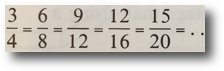These can be represented on a number line, all sharing the same position.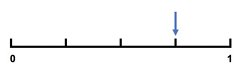Children can draw in the smaller marked positions between each quarter for each equivalent fraction and look for patterns.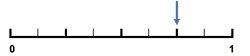These set of equivalences can also be written as: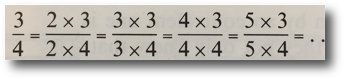So the numerator and denominator have both been multiplied by 2, then 3, 4, 5... and so on.

Given plenty of opportunities to explore equivalent fractions, children will come to understand that there are an infinite number of equivalent fractions, but the ratio between the numerator and denominator always stays the same.

### Proportional reasoning

Exploring different equivalent fractions is all about giving opportunities for proportional reasoning. Try these starting points:

• Continue and describe the pattern. What do you notice?

2/3 = 4/6 = 6/9 = 8/12 = ...

• What is the same? What is different?

3/5      6/10

• Give me an easy equivalent fraction to 3/4. Now give a hard one. What makes it easy or hard?

• Give me an equivalent fraction to 4/5, and another, and another, and another… What do you notice?

• Is it always, sometimes or never true that equivalent fractions share the same position on a number line. How can you prove this?

• Which is the odd one out? Why?

3/10      6/30      30/100
Website design by SiteBuilder Bespoke